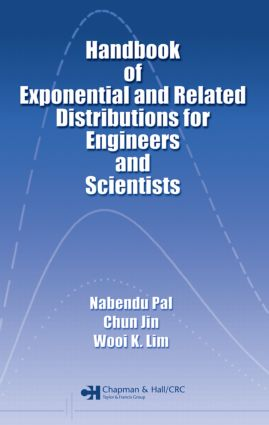# Handbook of Exponential and Related Distributions for Engineers and Scientists

## 1st Edition

Chapman and Hall/CRC

376 pages | 88 B/W Illus.

##### Purchasing Options:\$ = USD
Hardback: 9781584881384
pub: 2005-11-21
SAVE ~\$35.00
Currently out of stock
\$175.00
\$140.00
x
eBook (VitalSource) : 9780429208348
pub: 2005-11-21
from \$28.98

FREE Standard Shipping!

### Description

The normal distribution is widely known and used by scientists and engineers. However, there are many cases when the normal distribution is not appropriate, due to the data being skewed. Rather than leaving you to search through journal articles, advanced theoretical monographs, or introductory texts for alternative distributions, the Handbook of Exponential and Related Distributions for Engineers and Scientists provides a concise, carefully selected presentation of the properties and principles of selected distributions that are most useful for application in the sciences and engineering.

The book begins with all the basic mathematical and statistical background necessary to select the correct distribution to model real-world data sets. This includes inference, decision theory, and computational aspects including the popular Bootstrap method. The authors then examine four skewed distributions in detail: exponential, gamma, Weibull, and extreme value. For each one, they discuss general properties and applicability to example data sets, theoretical characterization, estimation of parameters and related inferences, and goodness of fit tests. The final chapter deals with system reliability for series and parallel systems.

Presenting methods based on statistical simulations and numerical computations, the Handbook of Exponential and Related Distributions for Engineers and Scientists supplies hands-on tools for applied researchers in need of practical tools for data analysis.

### Table of Contents

Acronyms

Notations

List of Figures

List of Tables

GENERAL STATISTICAL THEORY

Basic Concepts

The System of Real Numbers

Some Useful Algebraic Results

Set Theory

Introduction to Probability Theory

Random Variables

Joint Probability Distributions

Moment Generating Function

Order Statistics

Characteristic Function

Some Common Probability Distributions

Discrete Distributions

Continuous Distributions

Some Limit Theorems

Concepts of Statistical Inference

Introduction

Sufficiency and Completeness

Methods of Estimation

Methods of Evaluating Estimators

Elements of Hypothesis Testing

Set (or Interval) Estimation

Elements of Decision Theory

Introduction

Optimality Criteria

Loss Functions

Admissible, Minimax and Bayes Rules

Computational Aspects

Preliminaries

Numerical Integration

Monte-Carlo Simulation

Bootstrap Method of Resampling

Testing and Interval Estimation Based on Computations

EXPONENTIAL AND OTHER POSITIVELY SKEWED DISTRIBUTIONS WITH APPLICATIONS

Exponential Distribution

Preliminaries

Characterization of Exponential Distribution

Estimation of Parameter(s)

Goodness of Fit Tests for Exponential Distributions

Gamma Distribution

Preliminaries

Characterization of Gamma Distribution

Estimation of Parameters

Goodness of fit Tests for Gamma Distribution

Weibull Distribution

Preliminaries

Characterization of Weibull Distribution

Estimation of Parameters

Goodness of Fit Tests for Weibull Distribution

Extreme Value Distributions

Preliminaries

Characterizations of Extreme Value Distributions

Estimation of Parameters

Goodness of Fit Tests for Extreme Value Distributions

System Reliability

Preliminaries

Single Component Systems

Reliability of a Series System with Componentwise Data

Reliability of a Parallel System with Componentwise Data

Bibliography

Selected Statistical Tables

Index

### Subject Categories

##### BISAC Subject Codes/Headings:
MAT029000
MATHEMATICS / Probability & Statistics / General